# Gradients in NMR Spectroscopy – Part 5: The Pulsed Gradient Spin Echo (PGSE) Experiment

Part 3 discussed how a matched pair of positive/negative magnetic field gradient pulses can be used to encode spins for their displacement. Although this simple sequence has great value from an educational point of view, it is rarely used in practice due to several drawbacks.

• The delay time between the two gradient pulses can be quite long (up to several hundreds of milliseconds or even seconds). During that time the spins acquire additional phase information due to chemical shift evolution. This will make it impossible to phase the spectrum if it contains more than one peak.
• During the evolution the magnetisation suffers from T2* relaxation, which can lead to significant signal attenuation.
• For this experiment to work, it is extremely important that the two gradient pulses are very well matched in length and amplitude. This is very difficult to achieve with a positive/negative pair.

In 1965, E.O. Stejskal and J.E. Tanner published a famous paper describing an experiment which avoids these issues. It is called the Pulsed Gradient Spin Echo (PGSE) experiment, and I love this experiment because it’s so simple, yet technically very challenging. It is still being used in its original form after being around for half a century. The basic idea is shown in Figure 1.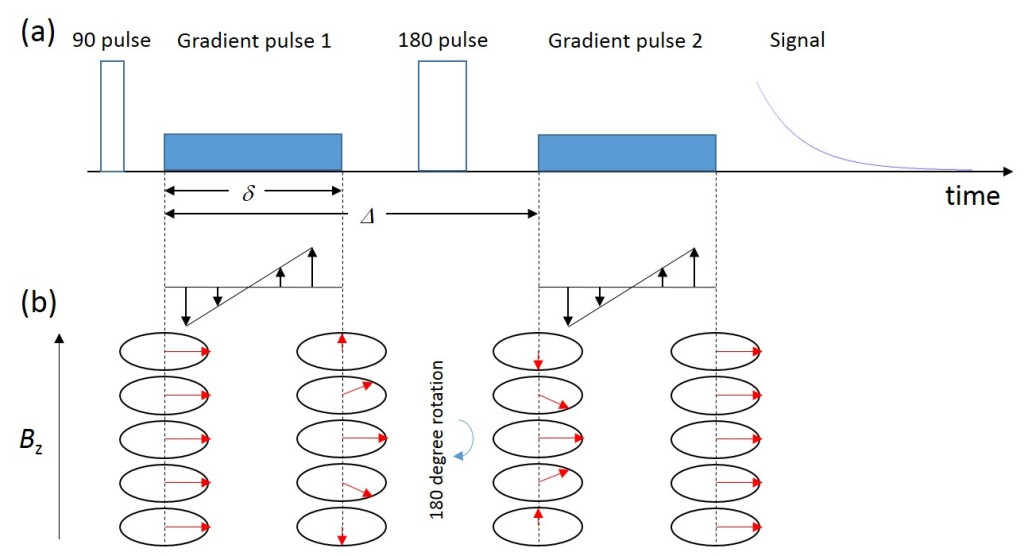Figure 1: (a) Schematic diagram of the PGSE pulse sequence. (b) The phase evolution of the spins at different locations along the gradient direction. Note that the 180 degree pulse inverts the phase wrap imposed by the first gradient pulse. The second gradient pulse, which is now identical to the first one in amplitude and length, completely refocuses this phase wrap.

The first thing that Stejskal and Tanner did was to insert a 180 degree pulse in the middle between the 90 degree pulse and the signal acquisition. This forms a spin echo and refocuses any chemical shift evolution at the start of the acquisition. The signal decay is now governed by T2, which is normally much longer than T2*. However, since the 180 degree pulse inverts the spin phases, the negative gradient pulse won’t refocus the signal any more. But here is the clever idea: By inverting the second gradient pulse from being negative to positive, we can refocus the phase wrap imposed by the first gradient completely, as shown in Figure 1 (b).

Stejskal and Tanner did a detailed mathematical analysis on the measured signal amplitude. For the case of self-diffusion of molecules (self-diffusion was the topic of part 4 in this series) they found that the normalised signal amplitude E/E0 decays as a Gaussian curve with increasing gradient pulse amplitude g: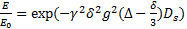(Equation 1)

In this equation, γ is the gyromagnetic ratio of the nucleus, δ and Δ are the duration and separation of the gradient pulses, and Ds the self-diffusion coefficient of the molecule. This sounds like a rather complicated and scary equation to deal with, but when an experiment is run, this is all calculated automatically. Normally, in order to measure the self-diffusion coefficient Ds one would run a series of experiments, where either the duration or the amplitude of the gradient pulses are incremented from one experiment to the next. Figure 2 below shows a series of stacked spectra of neat cyclohexane with increasing gradient amplitude. As the gradient increases, the peak height goes down. These data were acquired on a Spinsolve benchtop NMR spectrometer with additional hardware to enable PFGs for measuring diffusion.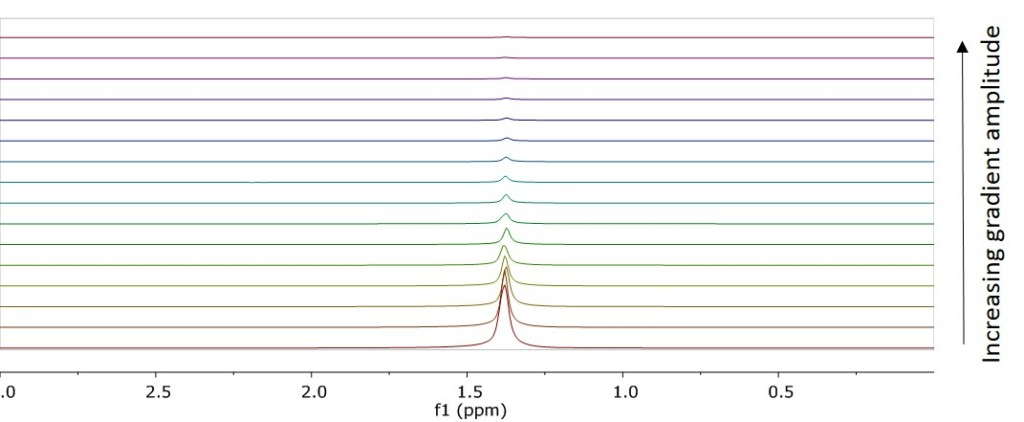Figure 2: A stacked plot of measured spectra of neat cyclohexane with increasing gradient amplitude.

Figure 3 (a) shows a plot of the peak integral versus the gradient amplitude (blue squares), along with a Gaussian fit of Ds according to equation 1 above (blue line). The fit curve matches the measured data pretty well, but from such a plot it is hard to see the quality of the fit, particularly for large gradient amplitudes, where the peak integral becomes very small. Mathematicians like to transform such plots so that the resulting curves turn into straight lines. For a PGSE data set such a transformation can be easily achieved by defining two new variables x and y in the following way:(Equation 2)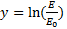(Equation 3)

The ln() function is the natural logarithm, which is the inversion of the exponential function. By combining equations 1, 2 and 3 we can re-write equation 1 as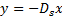(Equation 4)

Equation 4 is now the equation for a straight line with the slope –Ds. This means that if we plot the natural logarithm of the normalised peak integral versus γ2δ2g2(Δδ/3), we should get a straight line with the slope –Ds. This way of plotting PGSE data goes right back to the original paper by Stejskal and Tanner and is commonly known as a Stejskal-Tanner plot. In Figure 3 (b) the data from (a) are displayed as a Stejskal-tanner plot. It is immediately clear that the measured data are all on a straight line.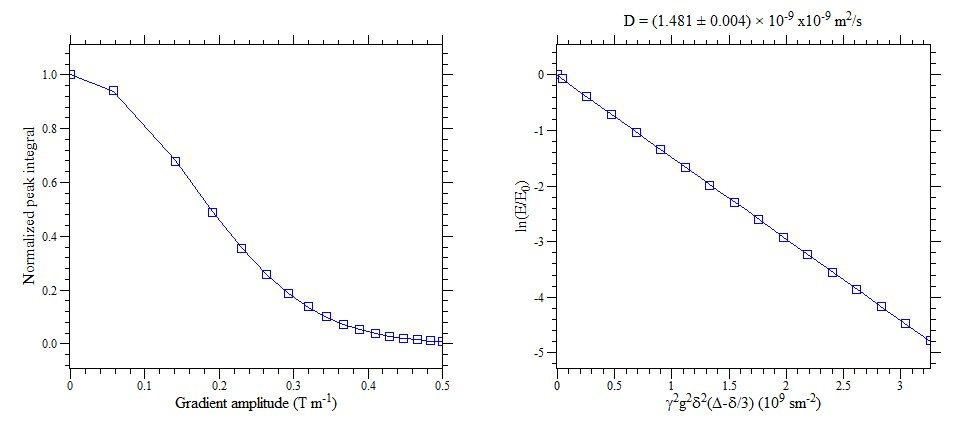Figure 3: (a) The peak integral from Figure 2 plotted versus the gradient amplitude g. (b) A better way to display PGSE data is the Stejskal-Tanner plot. For a single diffusion coefficient this should be a straight line with the negative slope being the self-diffusion coefficient.

There are many cases where the Stejskal-tanner plot does not form a straight line. An example is shown in Figure 4. These data were taken on a sample of commercial silicon oil. Looking at the plot of peak integral versus gradient amplitude, we notice small differences between measured data (red squares) and Gaussian fit according to equation 1 (red line). However, as these differences appear to be very subtle in this graph, we might be tempted to ignore them, or attribute them to some sort of artefact. But the Stejskal-Tanner plot of the same data reveals the poor quality of the fit. It is obvious that the single Gaussian model cannot describe these data, but we seem to have a distribution of self-diffusion coefficients in this case. In part 4 of this series we found that the self-diffusion coefficient can serve as a probe of the size of the molecule that is measured. So the distribution in diffusion coefficients that we see here indicates that the oil sample consists of molecules with a distribution of chain lengths.

Obviously this oil consists of molecules with a distribution of chain lengths.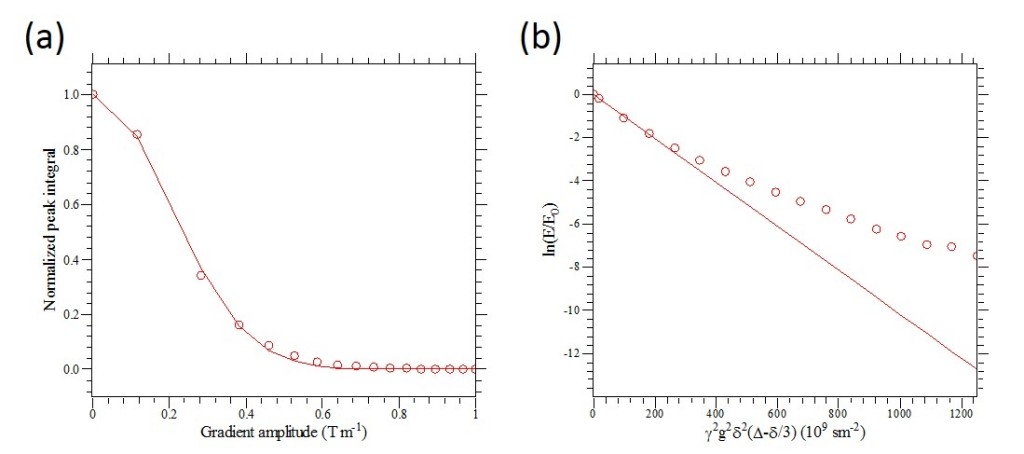Figure 4: (a) The peak integral from a sample of silicon oil plotted versus the gradient amplitude g. The fitted line does not look too bad in this graph. (b) The same data displayed as a Stejskal-Tanner plot, where the deviation from the straight line is strikingly obvious.

This has all been quite technical. The messages that I want to emphasize in this post are the following:

• The PGSE experiment is a simple, but very powerful technique to measure self-diffusion.
• The Stejskal-Tanner plot is a great way for displaying PGSE data.

Please stay tuned for the next post in this series, I promise it will be less technical. We will discuss the DOSY experiment – a nice way to separate spectral components in a mixture.